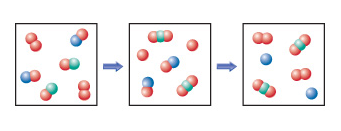# Problem: The following diagram represents an imaginary two-step mechanism. Let the red spheres represent element A, the green ones element B, and the blue ones element C.Write the equation for the net reaction that is occurring.

###### FREE Expert Solution

We have to write an equation for the net reaction using the given depiction of a chemical reaction.

We have the following information:

A = red spheres

B = green spheres

C = blue spheres###### Problem Details

The following diagram represents an imaginary two-step mechanism. Let the red spheres represent element A, the green ones element B, and the blue ones element C.Write the equation for the net reaction that is occurring.